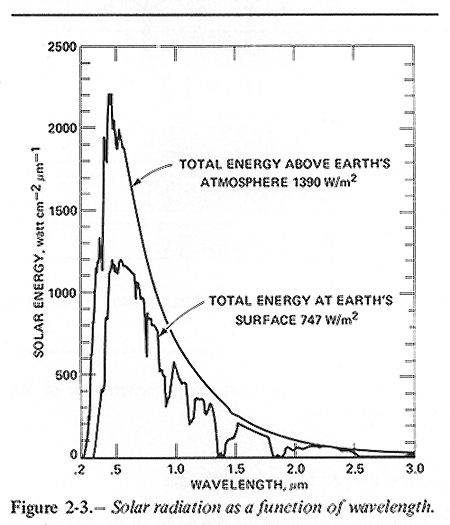# The number of photons ejected from the sun in a second

How many photons does the sun emit in a second?

The following worksheet was used in companion with this post for chemistry students.

Our chemistry class used the work of Brian Cox and (originally) John Herschel to determine the number of photons ejected from the sun in one second.

In this video, Brian Cox, repeats John Herschel’s famous experiment in Death Valley to determine the amount of energy the sun transmits to a given square meter of land.

From here, if you know the distance of the earth from the center of the sun, you can calculate the total amount of energy the sun emits in any direction by imaging the area of the sphere with this radius and multiplying by the amount of energy that heat up the can in one second.

The amount of energy delivered to the can is a type of calorimetry experiment:${E_{water}}\approx m_w\cdot{c_w}\cdot\Delta{T} = 1 \frac{kW}{m^2}\cdot{area}\cdot{time}$.

The total energy from the sun at this diameter is calculated using:${E_{total}} = 1 \frac{kW}{m^2}\cdot{4\pi\left({150\times{10^9}m}\right)^2}\cdot 1s = 2.8 \times 10^{26} J$.

Now, if we can estimate the energy of the average photon, we can estimate the number of photons this is.${E_{total}} = E_{photon}*N_{photons}$

Using this image (also below) of the distribution of photon wavelengths (obtained from a very interesting NASA space settlements website), we can estimate the “typical” wavelength of light coming from the sun.The class estimated this to be between 0.7 and 0.8 microns or$7.5 \times 10^{-7} m$

We know that the energy of a photon can be calculated according to$E_{photon} = \frac{hc}{\lambda}$.

So there are approximately:$N = \frac{2.8 \times 10^{26} J}{\frac{hc}{\lambda}}= \frac{\left(2.8 \times 10^{26} J\right)\cdot\left({7.5} \times 10^{-7} m\right)}{\left(6.626\times10^{-34}J\cdot{s}\right)\cdot\left({3.0 \times 10^{8}}\frac{m}{s}\right)} \approx 1\times10^{45}$ photons leaving the sun every second!

The same kind of modeling (flux analysis) could be applied to determine the relative amount of energy that reaches other planets in the solar system (and thus exactly how much colder earth would be if it were twice as far away. The answer is that number of photons would scale as$\frac{1}{R^2}$, so it would be four times colder!).

This analysis would also apply to stars that we view across the universe!

So the next question is, “How do we distinguish dimmer stars that are closer from brighter stars that are further away?”

The following worksheet can be used in companion with this material for multivariable calculus students.

Now that would be an interesting project for a multivariable calculus or an advanced chemistry student!I am a math and science teacher at a boarding school in Delaware.

Tagged with: ,
Posted in Advanced Chemistry, Multivariable Calculus
###### 4 comments on “The number of photons ejected from the sun in a second”
1.Peter Signell says:

It would be nice to have either (1) the time that Brian Cox observed for a 1K increase in temperature; or (2) the time speed-up factor resulting from time-lapse photography in the scene showing the thermometer. Either would allow students to do the math without having to go to Death Valley or to reverse-engineer the experiment!

2. […] Sun is roughly typical star and emits about photon per second in the visible range. It takes about 100 photon incident on the pupil, of […]

3.Matthew Moreland James says:

I just did this rough calculation using the number of photons needed for the human eye to detect a light source, when replying to a YouTube comment, and I came to a similar answer.

“+Silicondoc Well, let’s do some research and calculations. First off, I have found that it takes at least about 10 photons within a 100 ms time period for our eyes/brain to register that we saw a flash of light (http://math.ucr.edu/home/baez/physics/Quantum/see_a_photon.html). And considering the human pupil is about 1 cm^2 (10^-4 m^2), we can say that in order to see a light source with the naked eye, there must be at minimum 100 photons per second going through an area of 1 cm^2 or 10^6 photons per second going through an area of 1 m^2.
Now, the farthest single star that can be seen with the naked eye is “V762 Cassiopeiae, which is just visible under dark skies and is around 16,300 light years away” (http://www.howitworksdaily.com/which-is-the-farthest-star-that-we-can-see/) [I know, somewhat shady source, but plausible]. This distance is obviously way less than the proposed 1 million light years in your post.
Now, all we have to do is calculate the surface area (in meters) of a sphere with a radius of 16,300 light years and multiply that by the minimum 10^6 photons per second going through an area of 1 m^2 to find the minimum number of photons the star has to be emitting per second. Luckily that is not too difficult.
The equation for the surface are of a sphere is A=4πR^2. In our case the radius R is 16,300 light years (which is about 1.5*10^20 meters). So the star must emit at least (4π(1.5*10^20)^2)*1000 or about 3*10^44 photons per second. And to error on the side of caution let’s increase that number by a factor of 100 to compensate for any rounding errors. So let’s say a star releases about 3*10^46 photons per second in the visible spectrum.

4. […] see the world around you by a process whereby the sun emits rays of light (a great read here: it emits around 1045 every second!), most of which go out in to space never to be seen again. A […]